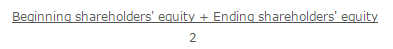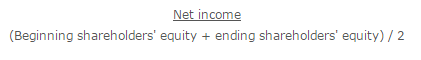# What is Average Shareholders Equity?

Average shareholders equity is an averaging concept used to smooth out the results of the return on equity calculation. This concept yields a more believable return on equity measurement.

The average shareholders equity calculation is the beginning shareholders equity plus the ending shareholders’ equity, divided by two. Thus, the formula is:The concept is most useful when measuring the return on investment in a period in which a business has sold a large amount of stock. In this case, the ending shareholders equity figure will be much higher than the beginning figure, which results in a substantially lower return on equity calculation. If there are few stock sales over time, this means that a trend line of return on equity measurements will reveal a sharp dip in any period in which stock is sold, even if the overall returns of the business are approximately the same over time.

The concept may be built directly into the return on equity formula, where the average is stated in the denominator, as follows:Average Shareholders Equity is a variation of the return on equity formula which calculates the shareholders’ equity component by adding shareholders’ equity at the beginning of a time period to the shareholders’ equity at the end of that time period and averaging the total.